# Introduction to Algebra - Multiplication

## A Puzzle

What is the missing number?

× 4 = 8

The answer is 2, right? Because 2 × 4 = 8.

Well, in Algebra we don't use blank boxes, we use a letter. So we might write:

 x × 4 = 8

But the "x" looks like the "×" ... that can be very confusing ... so in Algebra we don't use the multiply symbol (×) between numbers and letters:

We put the number next to the letter to mean multiply:

 4x = 8

In English we say "four x equals eight", meaning that 4 x's make 8.

 x = 2

## How to Solve

Instead of saying "obviously x=2", use this neat step-by-step approach:

• Work out what to remove to get "x = ..."
• Remove it by doing the reverse
• Do that to both sides

And what is the reverse of multiplying? Dividing!

Have a look at this example:

We want
to remove
the "4"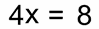To remove it, do
the reverse
, in
this case divide by 4Do it to
both sidesWhich is ...Solved!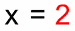## Why did we divide by 4 on both sides?

### Because of the need for balance ...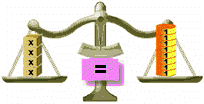In Balance
 Divide Left by 4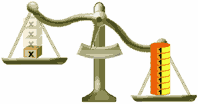Out of Balance!
 Divide Right by 4 Also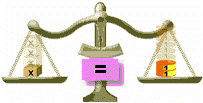In Balance Again

### Just remember ...

 To keep the balance, what we do to one side of the "=" we should also do to the other side!

## Another Puzzle

Solve this one:
 x / 3 = 5

What we want is an answer like "x = ...", but the divide by 3 is in the way of that!

If we multiply by 3 we can cancel out the divide by 3 (because 3/3=1)

So, let us try multiplying by 3 on both sides:x3 ×3 = 5 ×3
A little arithmetic (13×3 = 1 and 5×3 = 15) becomes:1x = 15
Which is just:x = 15
Solved!
(Quick Check: 15/3 = 5)

## More Complicated Example

How do we solve this?

 x / 3 + 2 = 5

It might look hard, but not if we solve it in stages.

First let us get rid of the "+2":

To remove the plus 2 use minus 2 (because 2−2=0) x/3 + 2 −2 = 5 −2
A little arithmetic (2−2 = 0 and 5−2 = 3) becomes:x/3 + 0 = 3
Which is just:x/3 = 3

Now, get rid of the "/3":

multiply by 3 to cancel out the divide by 3: x/3 ×3 = 3 ×3
A little arithmetic (3/3 = 1 and 3×3 = 9) becomes:1x = 9
Which is just:x = 9
Solved!
(Quick Check: 9/3 + 2 = 3+2 = 5)

## When you get more experienced:

When you get more experienced, you can solve it like this:

Subtract 2 from both sides: x/3 + 2 −2 = 5 −2
Simplify:x/3 = 3
Multiply by 3 on both sides: x/3 ×3 = 3 ×3
Simplify:x = 9
Or faster like this:
Subtract 2 from both sides:x/3 = 3
Multiply by 3: x = 9

## Real World Example### Example: Sam bought 3 boxes of chocolates online. Postage was \$9 and the total cost was \$45. How much was each box?

Let's use x for the price of each box.

3 times x plus \$9 is \$45:

3x + 9 = 45

Let's solve!

Subtract 9 from both sides:3x + 9 − 9 = 45 − 9
Simplify:3x = 36
Divide by 3:3x /3 = 36 /3
Simplify:x = 12

So each box was \$12

Advanced: we can also do the "divide by 3" first (but we must do it to all terms):

Divide by 3:3x/3 + 9/3 = 45/3
Simplify:x + 3 = 15
Subtract 3 from both sides:x + 3 − 3 = 15 − 3
Simplify:x = 12